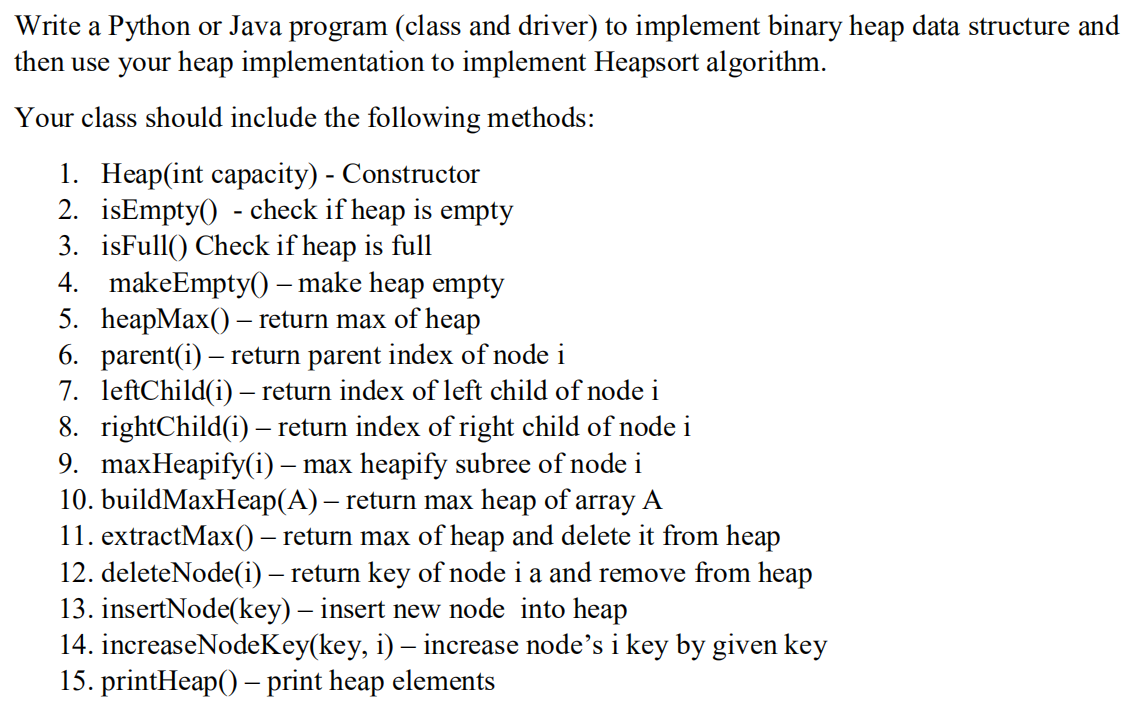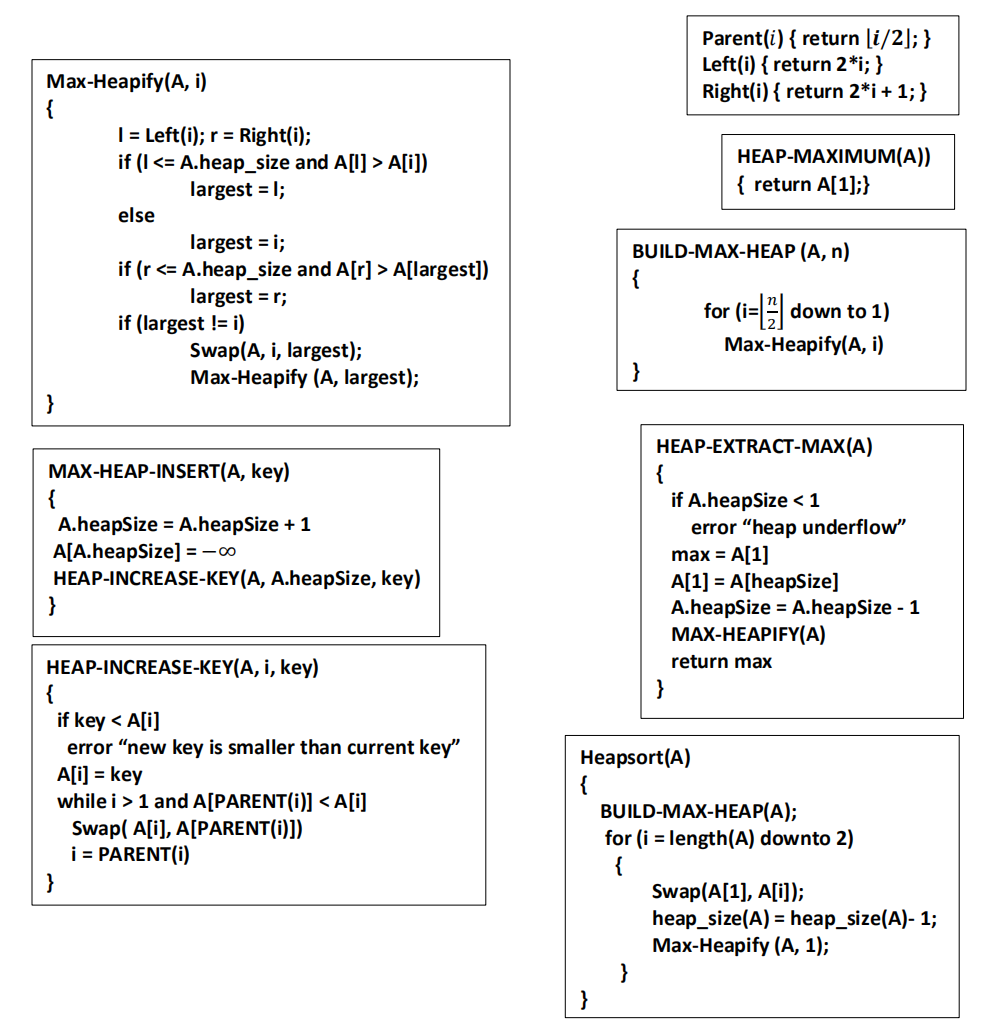# (Solved) : Write Python Java Program Implement Binary Heap Data Structure Use Heap Implementation Imp Q42710295 . . .

Write a Python or Java programto implement binary heap data structure and

then use your heap implementation to implement Heapsortalgorithm.Write a Python or Java program (class and driver) to implement binary heap data structure and then use your heap implementation to implement Heapsort algorithm. Your class should include the following methods: 1. Heap(int capacity) – Constructor 2. isEmpty() – check if heap is empty 3. isFull() Check if heap is full 4. makeEmpty() – make heap empty 5. heapMax() – return max of heap 6. parent(i) – return parent index of node i 7. leftChild(i) – return index of left child of node i 8. rightChild(i) – return index of right child of node i 9. max Heapify(i) – max heapify subree of node i 10. buildMaxHeap(A) – return max heap of array A 11. extractMax() – return max of heap and delete it from heap 12. deleteNode(i) – return key of node i a and remove from heap 13. insertNode(key) – insert new node into heap 14. increaseNodeKey(key, i) – increase node’s i key by given key 15. printHeap() – print heap elements Parent(i) { return [i/2]; } Left(i) { return 2*i; } Right(i) { return 2*i + 1;} Max-Heapify(A, i) HEAP-MAXIMUM(A)) { return A;} I = Left(i); r = Right(i); if (i <= A.heap_size and A[l] > A[i]) largest = 1; else largest = 1; if (r <= A.heap_size and A[r] > A[largest]) largest = r; if (largest != i) Swap(A, i, largest); Max-Heapify (A, largest); BUILD-MAX-HEAP (A, n) { for (i=1*| down to 1) Max-Heapify(A, i) HEAP-EXTRACT-MAX(A) MAX-HEAP-INSERT(A, key) A.heapSize = A.heapSize + 1 A[A.heapSize) = -00 HEAP-INCREASE-KEY(A, A.heapSize, key) if A.heapSize < 1 error “heap underflow” max = A A = A[heapSize] A.heapSize = A.heapSize – 1 MAX-HEAPIFY(A) return max HEAP-INCREASE-KEY(A, i, key) Heapsort(A) if key <A[i] error “new key is smaller than current key” A[i] = key while i > 1 and A[PARENT(0)] <A[i] Swap( A[i], A[PARENT(0)]) i = PARENT(0) BUILD-MAX-HEAP(A); for (i = length(A) downto 2) Swap(A, A[i]); heap_size(A) = heap_size(A)- 1; Max-Heapify (A, 1); Show transcribed image text Write a Python or Java program (class and driver) to implement binary heap data structure and then use your heap implementation to implement Heapsort algorithm. Your class should include the following methods: 1. Heap(int capacity) – Constructor 2. isEmpty() – check if heap is empty 3. isFull() Check if heap is full 4. makeEmpty() – make heap empty 5. heapMax() – return max of heap 6. parent(i) – return parent index of node i 7. leftChild(i) – return index of left child of node i 8. rightChild(i) – return index of right child of node i 9. max Heapify(i) – max heapify subree of node i 10. buildMaxHeap(A) – return max heap of array A 11. extractMax() – return max of heap and delete it from heap 12. deleteNode(i) – return key of node i a and remove from heap 13. insertNode(key) – insert new node into heap 14. increaseNodeKey(key, i) – increase node’s i key by given key 15. printHeap() – print heap elements
Parent(i) { return [i/2]; } Left(i) { return 2*i; } Right(i) { return 2*i + 1;} Max-Heapify(A, i) HEAP-MAXIMUM(A)) { return A;} I = Left(i); r = Right(i); if (i A[i]) largest = 1; else largest = 1; if (r A[largest]) largest = r; if (largest != i) Swap(A, i, largest); Max-Heapify (A, largest); BUILD-MAX-HEAP (A, n) { for (i=1*| down to 1) Max-Heapify(A, i) HEAP-EXTRACT-MAX(A) MAX-HEAP-INSERT(A, key) A.heapSize = A.heapSize + 1 A[A.heapSize) = -00 HEAP-INCREASE-KEY(A, A.heapSize, key) if A.heapSize

Answer to Write a Python or Java program to implement binary heap data structure and then use your heap implementation to implemen…

We are the best freelance writing portal. Looking for online writing, editing or proofreading jobs? We have plenty of writing assignments to handle.